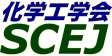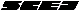## \$B9V1i%W%m%0%i%`!J%;%C%7%g%sJL!K(B

### \$B%7%s%]%8%&%`(B \$B!cJ,N%%W%m%;%9It2q%]%9%?!<%;%C%7%g%s!d(B

#### XA2P01-XA2P40

 \$B%*!<%,%J%\$%6!<(B \$B@V>>(B \$B7{

\$BJ,N%%W%m%;%9\$K4X\$9\$k4pAC\$+\$i1~MQ8&5f\$^\$GI}9-\$/%]%9%?!4\$r9T\$\$\$^\$9!%(B

\$B:G=*99?7F|;~!'(B2012-08-20 15:02:17
\$B9V1i(B
\$B;~9o(B
\$B9V1i(B
\$BHV9f(B
\$B9V1iBjL\!?H/I=\$B%-!<%o!<%I(B\$BJ,N`(B
\$BHV9f(B
\$B\$BHV9f(B
XA\$B2q>l(B \$BBh(B2\$BF|(B
9:00\$B!A(B 12:00XA2P01\$B%\$%*%s%S!<%`>H
(\$Bk(B \$BBg8J(B \$B!&(B (\$B3X(B)\$B>.Ln(B \$BN5J?(B \$B!&(B \$BO8c(B \$B!&(B Bukhari Abudullah \$B!&(B (\$B868&NL;R%S!<%`(B) \$B?yK\(B \$B2m \$B!&(B \$B5H@n(B \$B@5?M(B \$B!&(B (JFCC) \$B:4!9LZ(B \$BM%5H(B \$B!&(B (\$B
MOR zeolite membrane
ion beam treatment
water/isopropanol
S-2320
9:00\$B!A(B 12:00XA2P02\$B6%Ah5[CeK!\$K\$h\$k?e;:GQ4~J*\$N%+%I%_%&%`=|5n(B
(\$BKLBg1!?e;:2J3X8&5f1!(B) \$B!{(B(\$B3X(B)\$B@>J?(B \$BIp;K(B \$B!&(B (\$B@5(B)\$B4X(B \$B=(;J(B \$B!&(B (\$B@5(B)\$B4];3(B \$B1QCK(B
squid liver
S-2359
9:00\$B!A(B 12:00XA2P03\$B%7%j%+J#9gKl\$N9b29Fs;@2=C:AGF)2aFC@-(B
(\$B>;3(B \$B3(H~(B \$B!&(B \$BFb3\$(B \$BX*2p(B \$B!&(B \$B9u>B(B \$BNI2p(B \$B!&(B \$B2OK\(B \$B9b;V(B \$B!&(B \$BCSED(B \$BJb(B \$B!&(B (\$B@5(B)\$BLnB<(B \$B4490(B
silica hybrid membrane
counter diffusion CVD
high temperature CO2 separation
S-23171
9:00\$B!A(B 12:00XA2P04\$BNPAt\$N?'AG%?%s%Q%/
(\$BKLBg1!@8L?(B) \$B!{(B(\$B3X(B)\$B8yEa(B \$B4p(B \$B!&(B \$B9bNS(B \$B8|;K(B \$B!&(B \$BEDCf(B \$BJb(B
Green alage
ClearNative-PAGE
S-23176
9:00\$B!A(B 12:00XA2P05\$BK"KwJ,N%K!\$K\$h\$kF}@6%?%s%Q%/
(\$BKLBg1!?e(B) \$B!{(B(\$B3X(B)\$B;3K\(B \$B9'Fs(B \$B!&(B (\$B@5(B)\$B4];3(B \$B1QCK(B \$B!&(B (\$B@5(B)\$B4X(B \$B=(;J(B
whey
protein
foam separation
S-23187
9:00\$B!A(B 12:00XA2P06\$B%j%s;i
(\$B?@8MBg1!9)(B) \$B!{(B(\$B3X(B)\$BC+K\(B \$BC\$Li(B \$B!&(B (\$B@5(B)\$B:4Gl(B \$BBgJe(B \$B!&(B (\$B@5(B)\$B_7ED(B \$BM&@8(B \$B!&(B (\$B@5(B)\$B>>;3(B \$B=(?M(B
Reverse osmosis membrane
Biofouling
Phospholipid polymer
S-23259
9:00\$B!A(B 12:00XA2P07\$B%7%j%+N3;R7|By1U\$ND62;GHL82=\$K\$h\$kN3;RJ,2h(B
(\$BL>Bg1!9)(B) \$B!{(B(\$B3X(B)\$B2,(B \$BD>NI(B \$B!&(B (\$B3X(B)\$BLnF~(B \$BMNN<(B \$B!&(B (\$B@5(B)\$BFs0f(B \$B?8(B
Ultrasonic atomization
SiO2 particle
Fractionation. Dispersion
S-23282
9:00\$B!A(B 12:00XA2P08\$BJ,;R\$U\$k\$\$C:AGKl\$N%9%k%[%s2=(B
(\$B;38}Bg1!M}9)(B) \$B!{(B(\$B3X(B)\$B@P@n(B \$BM598(B \$B!&(B (\$B3X(B)\$B8E2l(B \$BCR;R(B \$B!&(B (\$B@5(B)\$B7'@Z(B \$B@t(B \$B!&(B (\$B@5(B)\$BEDCf(B \$B0l9((B \$B!&(B (\$B@5(B)\$B4nB?(B \$B1QIR(B
carbon membrane
pervaporation
surface modification
S-23301
9:00\$B!A(B 12:00XA2P09\$BKl\$rMQ\$\$\$?N3;RJ,5iK!\$K\$*\$1\$kEE2r
(\$B9)3X1!Bg9)(B) \$B!{(B(\$B3X(B)\$BbCJI(B \$BNI(B \$B!&(B (\$B@5(B)\$B@V>>(B \$B7{ \$B!&(B (\$B@5(B)\$BCfHx(B \$B??0l(B
Membrane
Classification
Electrolyte
S-23307
9:00\$B!A(B 12:00XA2P10\$B%a%=%]!<%i%9%7%j%+(B/\$B%]%j%\$%_%IJ#9gKl\$NF)2aJ*@-I>2A(B
(\$B;38}Bg1!M}9)(B) \$B!{ \$B!&(B (\$B@5(B)\$B7'@Z(B \$B@t(B \$B!&(B (\$B@5(B)\$BEDCf(B \$B0l9((B \$B!&(B (\$B@5(B)\$B4nB?(B \$B1QIR(B
CO2 separation
mesoporous silica
S-23313
9:00\$B!A(B 12:00XA2P11Y\$B7?%<%*%i%\$%HKl\$NF)2aJ*@-(B
(\$B;38}Bg1!M}9)(B) \$B!{(B(\$B3X(B)\$BCf@n(B \$B7DI'(B \$B!&(B (\$B@5(B)\$B7'@Z(B \$B@t(B \$B!&(B (\$B@5(B)\$BEDCf(B \$B0l9((B \$B!&(B (\$B@5(B)\$B4nB?(B \$B1QIR(B
zeolite membrane
pervaporation
organic liquid mixture
S-23317
9:00\$B!A(B 12:00XA2P12\$B:.9g%V%?%s!&%(%?%N!<%k!&?e7O9b05AjJ?9UJ*@-\$K4X\$9\$k4pAC8&5f(B
(\$BL>Bg1!9)(B) \$B!{(B(\$B3X(B)\$B>>B<(B \$B7rB@O:(B \$B!&(B (\$B@5(B)\$BD.ED(B \$BMN(B \$B!&(B (\$B@5(B)\$BKYE:(B \$B9@=S(B
vapor liquid equilibrium
butane
ethanol
S-23367
9:00\$B!A(B 12:00XA2P13Wet Process\$B\$K\$h\$C\$FD4@=\$5\$l\$?6bB0%J%NN3;R\$rMQ\$\$\$?N3;R@QAX7?L55!(BUF\$BKl\$N3+H/(B
(\$B?@8MBg1!9)(B) \$B!{(B(\$B3X(B)\$B2OED(B \$B?8<#(B \$B!&(B (\$B@5(B)\$B:4Gl(B \$BBgJe(B \$B!&(B (\$B@5(B)\$B?@Hx(B \$B1Q<#(B \$B!&(B (\$B@5(B)\$B>>;3(B \$B=(?M(B
layer-by-layer
Nanoparticles
membrane
S-23421
9:00\$B!A(B 12:00XA2P14\$B%U%)%H%j%=%0%i%U%#!<\$K\$h\$k%Q%i%8%&%`J#9gKl\$N:n@=\$HI>2A(B
(\$B4tIlBg1!9)(B) \$B!{(B(\$B3X(B)\$B86ED(B \$B??8c(B \$B!&(B (\$B3X(B)\$B:4F#(B \$B@?;J(B \$B!&(B (\$B4tIlBg9)(B) (\$B@5(B)\$B5\K\(B \$B3X(B \$B!&(B (\$B4tIlBg@8L?%;(B) (\$B@5(B)\$B6a9>(B \$BLwB'(B \$B!&(B (\$B4tIlBg9)(B) (\$B@5(B)\$B>e5\(B \$B@.G7(B \$B!&(B (\$BL>Bg1!9)(B) (\$B@5(B)\$B;3ED(B \$BGn;K(B \$B!&(B (\$B@5(B)\$BED@n(B \$BCRI'(B
Pd membrane
Photolithography
Hydrogen separation
S-23446
9:00\$B!A(B 12:00XA2P15\$B%^%\$%/%m%P%V%k\$K\$h\$k(BO/W\$B%(%^%k%7%g%sJ,N%\$K5Z\$\\$9L}E)
(\$BL>Bg1!9)(B) \$B!{(B(\$B3X(B)\$B8eF#(B \$B9,9((B \$B!&(B (\$B@5(B)\$B0BED(B \$B7<;J(B
emulsion
microbubble
zeta potential
S-23450
9:00\$B!A(B 12:00XA2P16EDTA-Sr, EDTA-Zn\$B\$H(BCu\$B\$H\$N6bB0CV49H?1~\$rMxMQ\$7\$?EE5\$F)@O\$K\$*\$1\$k(BSr, Zn\$B\$NJ,N%FC@-(B
(\$B=)EDBg1!9)3X;q8;(B) \$B!{(B(\$B@5(B)\$B9b66(B \$BGn(B \$B!&(B \$BBc(B \$B7CM\$(B \$B!&(B (\$B@5(B)\$B5FCO(B \$B8-0l(B
Sr
EDTA
Electrodialysis
S-23549
9:00\$B!A(B 12:00XA2P17\$B%O%\$%I%l!<%H\$r8GDjAj\$H\$9\$k%,%9%/%m%^%HJ,N%\$N8!F\$(B
(\$B;:Am8&(B) \$B!{(B(\$B@5(B)\$B;3K\(B \$B2B9'(B \$B!&(B (\$B@5(B)\$B@nB<(B \$BB@O:(B \$B!&(B (\$B@5(B)\$BDX(B \$BBnLi(B \$B!&(B (\$BElBg(B) (\$B@5(B)\$BJ?NS(B \$B?B0lO:(B \$B!&(B (\$BF|Bg(B) (\$B3X(B)\$BB \$B!&(B (\$B3X(B)\$BC]Fb(B \$B4p(B \$B!&(B (\$B@5(B)\$BJ]2J(B \$B5.N<(B \$B!&(B (\$B@5(B)\$BDT(B \$BCRLi(B
hydrate
gas chromatography
TBAB
S-23630
9:00\$B!A(B 12:00XA2P18\$BHyN3;RJ,;67O\$NKl:Y9&FbF)2a5sF0\$K4X\$9\$kD>@\?tCM%7%_%e%l!<%7%g%s(B
(\$B?@8MBg1!9)(B) \$B!{(B(\$B3X(B)\$BI[;\(B \$B\$R\$m\$_(B \$B!&(B (\$B@5(B)\$B@P?@(B \$BE0(B \$B!&(B (\$B;:5;C;Bg(B) \$B@uHx(B \$B?50l(B \$B!&(B (\$B?@8MBg1!9)(B) (\$B@5(B)\$B:4Gl(B \$BBgJe(B \$B!&(B (\$B@5(B)\$BBg8~(B \$B5H7J(B \$B!&(B (\$B@5(B)\$B?@Hx(B \$B1Q<#(B \$B!&(B (\$B@5(B)\$B>>;3(B \$B=(?M(B
Direct numerical simulation
trans membrane pressure
constant rate filtration
S-23668
9:00\$B!A(B 12:00XA2P19\$BCf6u;eKl@.7A\$K5Z\$\\$99bJ,;RMO1U\$NHs%K%e!<%H%sFC@-\$H8G2=B.EY\$N1F6A(B
(\$B?@8MBg1!9)(B) \$B!{(B(\$B3X(B)\$BAlC+(B \$BPR5#(B \$B!&(B (\$B@5(B)\$B@P?@(B \$BE0(B \$B!&(B (\$B@5(B)\$BBg8~(B \$B5H7J(B \$B!&(B (\$B@5(B)\$B:4Gl(B \$BBgJe(B \$B!&(B (\$B@5(B)\$B?@Hx(B \$B1Q<#(B \$B!&(B (\$B@5(B)\$B>>;3(B \$B=(?M(B
hollow fiber membrane
non-solvent induced phase separation
Barus effect
S-23699
9:00\$B!A(B 12:00XA2P20\$B30It\$HG.8r49\$N\$"\$kG(\$lJI>xN1Ec\$K\$*\$1\$kG.!&J*
(\$BL>9)Bg(B) \$B!{(B(\$B3X(B)\$B>!Ln(B \$BC\$Lo(B \$B!&(B (\$B@5(B)\$B?9(B \$B=( \$B!&(B (\$B@5(B)\$B4dED(B \$B=\$0l(B \$B!&(B \$BB< \$B!&(B \$BM{(B \$B_=h_(B
falling film
heat transfer
mass transfer
S-23729
9:00\$B!A(B 12:00XA2P21RHO\$B7?%<%*%i%\$%H(B/\$BAB?e@-%7%j%+J#9gKl\$K\$h\$kFs;@2=C:AG\$NA*BrJ,N%(B
(\$B4X@>Bg4D6-ET;T9)(B) \$B!{(B(\$B3X(B)\$B?yED(B \$BN4@8(B \$B!&(B (\$B@5(B)\$B9SLZ(B \$BDgIW(B \$B!&(B (\$B@5(B)\$B;3K\(B \$B=(
carbon dioxide
zeolite rho
hydrophobic membrane
S-23743
9:00\$B!A(B 12:00XA2P22\$B%0%i%_%7%8%s(BA\$B%A%c%M%k\$rMQ\$\$\$?%j%s;i
(\$B?@8MBg1!9)(B) \$B!{(B(\$B3X(B)\$B;32<(B \$B9d(B \$B!&(B (\$B@5(B)\$B:4Gl(B \$BBgJe(B \$B!&(B (\$B@5(B)\$BF#0f(B \$B><9((B \$B!&(B (\$B3X(B)\$B2qDE(B \$B?4G7N<(B \$B!&(B (\$B@5(B)\$B?@Hx(B \$B1Q<#(B \$B!&(B (\$B@5(B)\$B>>;3(B \$B=(?M(B
Reverse Osmosis
Liposome
Water channel
S-23758
9:00\$B!A(B 12:00XA2P23\$B%-%c%j%"M"Aw5!G=\$rM-\$9\$k%\$%*%s%2%kKl\$NAO@=\$K4X\$9\$k4pACE*8!F\$(B
(\$B?@8MBg1!9)(B) \$B!{(B(\$B3X(B)\$B3^86(B \$B>)J?(B \$B!&(B (\$B@5(B)\$B?@Hx(B \$B1Q<#(B \$B!&(B \$BFn(B \$BNh;R(B \$B!&(B (\$B@5(B)\$B>>;3(B \$B=(?M(B
Facilitated transport membrane
Gas separation
Ion gel
S-23795
9:00\$B!A(B 12:00XA2P24\$B5mJ5\$N0!NW3&?eG.=hM}@8@.J*\$rMQ\$\$\$?%9%H%m%s%A%&%`5[CeK!\$N3+H/(B
(\$B9qN)9q:]0eNE8&%;(B) \$B!{(B(\$B@5(B)\$B8~;3(B \$B9,;0(B \$B!&(B (\$B@5(B)\$B2VED(B \$B;0;MO:(B \$B!&(B (\$B@5(B)\$B;3K\(B \$B7rFs(B
cow dung powder
strontium
S-23803
9:00\$B!A(B 12:00XA2P25Y\$B7?%<%*%i%\$%HKl\$K\$h\$k(BIPA-H2O\$B>x5\$\$NO"B32sJ,C&?e(B
(\$B1'ET5\Bg9)(B) \$B!{(B(\$B3X(B)\$B@PED(B \$B=a(B \$B!&(B (\$B1'ET5\Bg1!9)(B) \$BBg_7(B \$B8,2p(B \$B!&(B (\$B@5(B)\$B:4F#(B \$B9d;K(B \$B!&(B (\$B@5(B)\$B0KF#(B \$BD>
Y type zeolite
dehydration
membrane
S-23814
9:00\$B!A(B 12:00XA2P26\$B6Q0l1U1UCj=PK!(B(HoLLE)\$B\$K\$h\$k1U>=%,%i%9Cf%\$%s%8%&%`\$NJ,N%!&G;=L\$H%j%5%\$%/%k\$X\$N2DG=@-(B
(\$B0q>kBg9)(B) \$B!{(B(\$B3X(B)\$B2CF#(B \$B7r(B \$B!&(B (\$B@5(B)\$B8^==Mr(B \$B=JO:(B \$B!&(B (\$B0q>k8)9)5;%;(B) (\$B@5(B)\$B@PEO(B \$B63G7(B \$B!&(B (\$B%Q!<%-%s%(%k%^!<%8%c%Q%s(B) \$B8E@n(B \$B??(B \$B!&(B (\$BJ*:`8&(B) \$B;38}(B \$B?N;V(B
Indium
Homogeneous Liquid-Liquid Extraction
HoLLE
S-23844
9:00\$B!A(B 12:00XA2P27\$BAjJ,N%\$rH<\$&:.9gMOG^7O%U%#%k%`\$N4%Ag%Q%9(B
(\$B6e9)Bg9)(B) \$B!{(B(\$B3X(B)\$BH*;3(B \$BH~5*;R(B \$B!&(B (\$B@5(B)\$B;3B<(B \$BJ}?M(B \$B!&(B (\$B@5(B)\$BGOEO(B \$B2B=((B \$B!&(B (\$B@5(B)\$B
Phase separation
Drying path
Coating
S-23850
9:00\$B!A(B 12:00XA2P28\$B%W%i%9%_%I(BDNA\$B\$N%"%U%#%K%F%#KlJ,N%K!\$N3+H/(B
(\$BL>Bg1!9)(B) \$B!{(B(\$B3X(B)\$BNkLZ(B \$B?rG7(B \$B!&(B (\$B@5(B)\$BJR6M(B \$B@?G7(B \$B!&(B (\$B@5(B)\$BF~C+(B \$B1Q;J(B
affinity membrane filtration
purification
plasmid DNA
S-23872
9:00\$B!A(B 12:00XA2P29\$B9bJ,;RKl\$K\$h\$k%J%NN3;R\$N<><0J,5i\$K4X\$9\$k4pAC8&5f(B
(\$BL>Bg9)(B) \$B!{(B(\$B3X(B)\$BGO(B \$BJ8@E(B \$B!&(B (\$B@5(B)\$B8~0f(B \$B9/?M(B
microfiltration membrane
nanoparticle
classification
S-23884
9:00\$B!A(B 12:00XA2P30\$B_I2a\$H5[Ce\$N5!G=\$rM-\$9\$k%O%\$%V%j%C%I%J%N%U%!%\$%P!
(\$BL>Bg9)(B) \$B!{(B(\$B3X(B)\$BE7Ln(B \$B1SG7(B \$B!&(B (\$B@5(B)\$B8~0f(B \$B9/?M(B
nanofiber
carbon nanoparticle
hybrid filter media
S-23886
9:00\$B!A(B 12:00XA2P31\$BJ.t5!9=\$rHw\$(\$?2sE>7?%@%\$%J%_%C%/%U%#%k%?!<\$N:GE,A`:n>r7o\$NC5:w(B
(\$BL>Bg9)(B) \$B!{(B(\$B3X(B)\$B>.NS(B \$B7rB@(B \$B!&(B (\$B@5(B)\$B8~0f(B \$B9/?M(B \$B!&(B (\$B=;M'EE5\$9)6H(B) \$BGp86(B \$B=( \$B!&(B \$B>e;3(B \$B=!Ih(B \$B!&(B \$BCf0f(B \$BN6;q(B
dynamic filter
constant rate filtration
optimization
S-23888
9:00\$B!A(B 12:00XA2P32\$B<+8JG.:F@87?3\$?eC8?e2=AuCV\$N3+H/(B
(\$BElBg(B) \$B!{(B(\$B3X(B)\$B?eLn(B \$B42G7(B \$B!&(B (\$B@5(B)\$B4Eht(B \$B \$B!&(B (\$B@5(B)\$B4_K\(B \$B7<(B \$B!&(B (\$B@5(B)\$BDi(B \$BFX;J(B
separation
desalination
energy saving
S-23933
9:00\$B!A(B 12:00XA2P33\$B%G%s%I%j%^!<(B/PVA\$B%O%\$%V%j%C%IKl\$N3+H/\$H(BCO2\$BJ,N%FC@-(B
(RITE) \$B!{(B(\$B@5(B)\$BCJ(B \$B=J9H(B \$B!&(B (\$B@5(B)\$B9CHe(B \$B>HI'(B \$B!&(B \$BC+8}(B \$B0iM:(B \$B!&(B (\$B@5(B)\$BIw4V(B \$B?-8c(B
dendrimer
CO2 separation
CCS
S-23937
9:00\$B!A(B 12:00XA2P34\$B3F2A(B
(\$B?@8MBg1!9)(B) \$B!{(B(\$B3X(B)\$BD9Eg(B \$BM@E5(B \$B!&(B (\$B@5(B)\$B:4Gl(B \$BBgJe(B \$B!&(B (\$B@5(B)\$B_7ED(B \$BM&@8(B \$B!&(B (\$B@5(B)\$B>>;3(B \$B=(?M(B
membrane material
biofilm
S-23938
9:00\$B!A(B 12:00XA2P35\$BM-5!!?L55!J#9gKl\$N3&LL9=B\$\$H%,%9F)2aFC@-(B
(\$BF`NI@hC \$B!&(B (RITE) (\$B@5(B)\$B@FF#(B \$B?r(B \$B!&(B \$BC+8}(B \$B0iM:(B \$B!&(B (RITE/\$BF`NI@hCHI'(B
organic/inorganic composite membrane
gas permeation
membrane structure
S-23945
9:00\$B!A(B 12:00XA2P36\$BAjJ,N%\$rMQ\$\$\$?GQ%0%j!<%9\$+\$i\$N%P%\$%*=EL}@=B\$\$H\$=\$N@->uI>2A(B
(\$B4D6-8&(B) \$B!{(B(\$B@5(B)\$BAR;}(B \$B=(IR(B \$B!&(B (\$BJ<8K8)Bg(B) (\$B@5(B)\$BA0ED(B \$B8w<#(B \$B!&(B (\$B@5(B)\$BJ!0f(B \$B7<2p(B \$B!&(B (\$B4D6-8&(B) \$BBgGw(B \$B@/9@(B
Waste grease
Biofuel
Phase separation
S-23952
9:00\$B!A(B 12:00XA2P37\$B%;%7%&%`9bB.=|5nMQ%U%'%m%7%"%s2=6bB0C4;}A!0]\$N:n@=(B
(\$B@iMUBg1!9)!&6&@81~2=(B) \$B!{(B(\$B3X(B)\$BE73\$(B \$BOK(B \$B!&(B (\$B3X(B)\$B2,B<(B \$BM:2p(B \$B!&(B \$BJ?;3(B \$BM:>M(B \$B!&(B \$BF#86(B \$BK.IW(B \$B!&(B (\$B4D6->t2=8&(B) \$B?\6?(B \$B9b?.(B \$B!&(B (\$B@iMUBg1!9)!&6&@81~2=(B) \$BG_Ln(B \$BB@Je(B \$B!&(B (\$B@5(B)\$B:XF#(B \$B630l(B
metal ferrocyanide
cesium removal
S-23954
9:00\$B!A(B 12:00XA2P38\$BMO=P%/%m%^%H\$G\$N9bJ,N%EY\$rC#@.\$9\$k\$?\$a\$N%]%j%^!<%V%i%7Ek:\N3;R\$N:n@=(B
(\$B@iMUBg9)(B) \$B!{(B(\$B3X(B)\$B?7=P(B \$B5s(B \$B!&(B (\$B3X(B)\$B@wC+(B \$B9'L@(B \$B!&(B (\$B3X(B)\$BAzED(B \$BM40l(B \$B!&(B \$BG_Ln(B \$BB@Je(B \$B!&(B (\$B@5(B)\$B:XF#(B \$B630l(B
protein purification
elution chromatography
plymer brush
S-23955
9:00\$B!A(B 12:00XA2P39\$B9b05(BCO2\$B2=3X5[<}1U\$N3+H/!=Hs?e7OMOG^\$NE:2C8z2L(B
(RITE) \$B!{(B(\$B@5(B)\$B;3K\(B \$B?.(B \$B!&(B \$BEl0f(B \$BN49T(B
Chemical CO2 Solvent
Non-aqueous Medium
High-pressure CO2
S-23962
9:00\$B!A(B 12:00XA2P40\$B?eAG5[B"9g6b\$rMQ\$\$\$??eAG4^M-%,%9\$+\$i\$N?eAG@:@=(B
(\$B?@8M@=9]=j(B) \$B!{(B(\$B@5(B)\$BF#_7(B \$B>4Mx(B \$B!&(B (\$B6&(B)\$BN1@n(B \$Bf{J?(B \$B!&(B (\$B6&(B)\$BC+8}(B \$B9T?-(B \$B!&(B (\$B@5(B)\$B;01:(B \$B??0l(B
Metal Hydride
Hydrogen Purification
S-231000

\$B9V1i%W%m%0%i%`(B
\$B2=3X9)3X2q(B \$BBh(B44\$B2s=)5(Bg2q(B(C) 2012 \$B8x1W
Most recent update: 2012-08-20 15:02:17
For more information contact \$B2=3X9)3X2qElKL;YIt(B \$BBh(B44\$B2s=)5(Bg2q(B \$BLd\$\$9g\$;78(B
E-mail: inquiry-44fwww3.scej.org
This page was generated byeasp 2.28; proghtml 2.28c (C)1999-2011 kawase